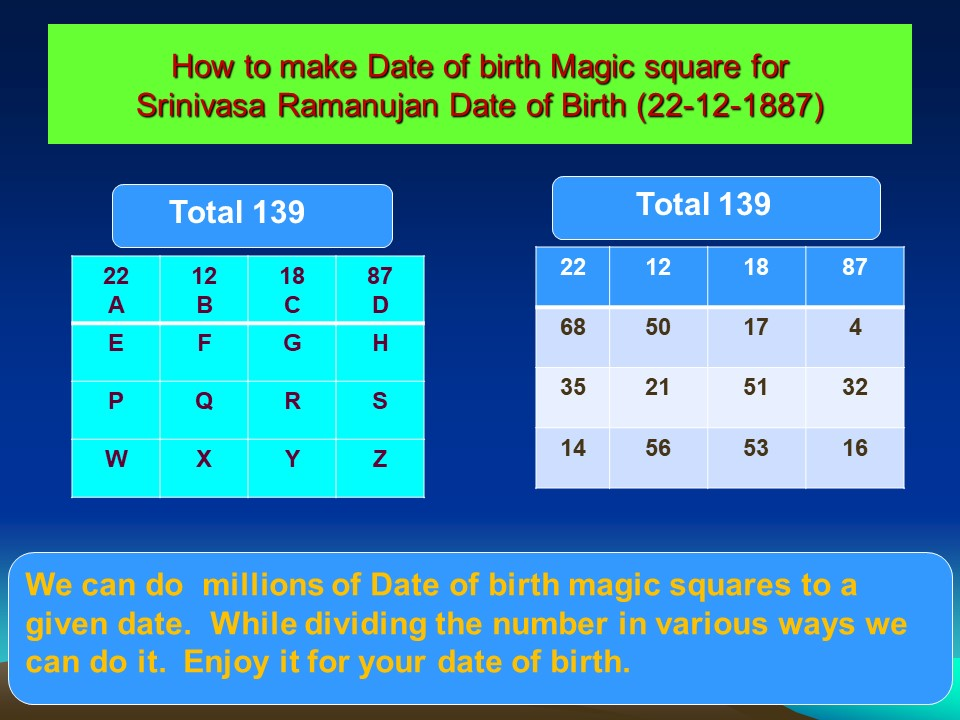# How to construct a Magic Square for a Given Date

Let us do magic square to the date of Birth of our Greatest  Indian Mathematician Sri Srinivasa Ramanujan.

His date of Birth is 22nd Dec 1887. The following is a Date-Of-Birth Magic Square with a Magic Total 139.

By following the given method we can do hundreds of, thousands of, millions of Date of Birth Magic Squares for any given date.

The following is a table with variables, which we will use in the explanation

#####1. Write the date in the top row first square, month in the second square and year in two parts in the third and fourth square.
2. Add all the four numbers and write on the top of the square (139).
3. Now we have to make a 4 x 4 magic square.
4. Draw a empty 4 x 4 square and replace 22 by A,   12 by B,   18 by C and 87 by D.
5. Now we know  A,B,C and D.   Rest of the values we have to find out.
6. Count all numbers in the total 1+3+9=13. Once again count  1+3=4. (add all numbers and make it a single digit. Write this in the H square
7. Now add B+C ( 12 + 18 =30). Divide 30 into two parts. i.e 14 and 16. Write 14 in W square and 16 in the Z square
8. By using the properties of Magic square, i.e all vertical, Horizontal and both Diagonal totals are equal, we are going to solve this Magic Square.
9. In the Fourth vertical column, we know the values of D,H,Z. we have to find Out the value of S. Hence S = 139 – (D+H+Z) = 139 — ( 87+4+16) = 139 – 107 = 32. This is the value of S. Write 32 in the S square
10. Now in Diagonals, we know the value of A and Z . Hence the value of F + R = 139 – (22 + 16) = 139-38= 101. Divide it into two parts 50 and 51 and write it in the F and R squares. F = 50 and R = 51.
11. In another diagonal, we know the value of D and W . Hence the value of  G+Q = 139 – (87 + 14) = 139-101= 38. Divide it into two parts 17 and 21 and write it in the F and R squares. G = 17 and Q = 21.
12. In the second row, we know the value of E,G,H. Hence value of E=139-(E+G+H)= 139 –( 50+17+4)= 139 – 71 = 68. Write 68 in E.
13. In the third row, we know the value of Q,R,S. Hence value of P=139-(Q+R+S)= 139 –(21+51+32)= 139 – 104 = 35. Write 35 in P.
14. Now in the second vertical column, we know the values of B,F,Q. Hence value of X = 139 – (12+50+21) = 139- 83 = 56. Write 56 in X
15. Now in the third vertical column, we know the values of C,G,R. Hence value of X = 139 – (18+17+51) = 139- 86 = 53. Write 53 in Y
16. Now add the values of W,X,Y and Z. You will  get 139 is the total.

Hurray!!! MAGIC SQUARE FOR 22-12-1887 IS READY

In step number 10, we have divided 101 into 50 and 51.  Instead it can be divided into q and 101 or 1 and 100 or 2 and 99 etc. etc… Interestingly we can divide it as -1 and 102 or -2 and 103 or -3 ans 104 etc.  While dividing each number, if the numbers are slightly changed, we can get a new Magic square.

Hence we can make hundreds of, thousands of, millions of date of birth magic squares for any given date

Enjoy Magic square for your date of Birth!!!    In various methods….

## 2 thoughts on “How to construct a Magic Square for a Given Date”

1.mini says:

hi
thanks for this update , but i am not able to figure out the points 10 and 11 .

in both there is mentioned – G+ Q .
plus there is a typo in point 4 – C should be 18 and not 19.

1.adminJollymathsBlog says: